# Cylindrical Function

## cylindrical function

[sə′lin·dri·kəl ‚fəŋk·shən]
(mathematics)

## Cylindrical Function

(or cylinder function, Bessel function). Cylindrical functions form a class of transcendental functions that is extremely important from the standpoint of applications in physics and engineering. These transcendental functions are solutions of the differential equationwhere v is an arbitrary parameter. Many problems of elastic, thermal, and electrical equilibrium and of the vibration of bodies of cylindrical shape reduce to this equation.

A solution of the form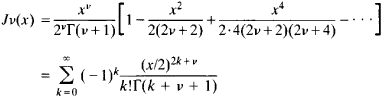is called a cylindrical function of order v of the first kind. Here, Γ(z) is the gamma function; the series on the right-hand side of the equation converges for all values of x. In particular, the zeroth-order cylindrical function has the form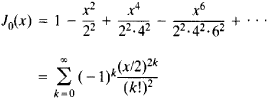If v is a negative integer (v = – n), then Jv(x) is defined in the following way:Cylindrical functions of order v = m + 1/2, where m is an integer, reduce to elementary functions—for example,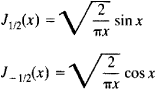The functions Jv(x) are often called simply Bessel functions, and equation (1) is known as the Bessel equation. These functions and equation (1), however, were used by L. Euler in a study of the vibrations of a membrane in 1766—that is, nearly 50 years before the work of F. Bessel. The zeroth-order function occurred still earlier in a paper by D. Bernoulli devoted to the oscillation of a heavy chain (published 1738), and the function of order 1/3 is found in a letter from Jakob Bernoulli to G. von Leibnitz (1703).

If v is not an integer, then the general solution of equation (1) has the form

(2) y = C1Jv(x) + C2Jv(x)

where C1 and C2 are constants. If, however, v is an integer, then Jv(x) and Jv(x) are linearly dependent, and their linear combination (2) is not a general solution of equation (1). For this reason, cylindrical functions of the second kind, or Neumann functions, were introduced: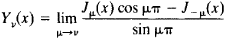With the aid of these functions the general solution of equation (1) may be written in the form

y = C1Jv(x) + C2Jv(x)

for both integral and nonintegral v.

In applications cylindrical functions of an imaginary argument are encountered: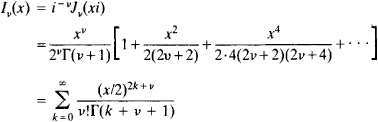(the modified cylindrical function of the first kind) and(the MacDonald function, or modified cylindrical function of the second kind). These functions satisfy the equationthe general solution of which has the form

y = C1Iv(x) + C2Kv(x

for both integral and nonintegral v.

Cylindrical functions of the third kind, or Hankel functions, are frequently used: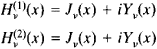The functions ber (x) and bei (x) are also often encountered. They are defined by the equation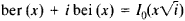An important role is played by asymptotic expressions of cylindrical functions for large values of the argument: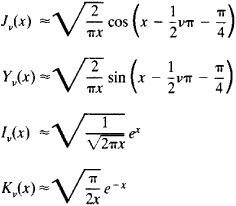It follows from these expressions in particular that the cylindrical functions Jv(x) and Yv(x) have an infinite set of real zeros arranged in such a way that far from the origin they are arbitrarily close to the zeros of the functions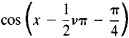and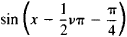respectively.

Cylindrical functions have been studied in great detail for complex values of the arguments. Many tables of cylindrical functions have been published for use in calculations.

### REFERENCES

Smirnov, V. I. Kurs vysshei matematiki, 8th ed., vol. 3, part 2. Moscow, 1969.
Nikiforov, A. F., and V. B. Uvarov. Osnovy teorii spetsial’nykh funktsii. Moscow, 1974.
Watson, G. N. Teoriia besselevykh funktsii, parts 1–2. Moscow, 1949. (Translated from English.)
Erdelyi, A., et al., eds. Vysshie transtsendentnye funktsii, 2nd ed., vol. 2. Moscow, 1974. (Translated from English.)
References in periodicals archive ?
For the first time, asymptotic representations of spectral Green's function components were heuristically obtained in  by combining the available plane-case formulas with the asymptotic cylindrical-case expressions for the two limiting relationships h [much grater than] n and h [much less than] n between cylindrical function index n and propagation constant h.
1,2]r) is the Bessel cylindrical function of the first kind m-th order; [[k.
perpendicular to]r]) is the first kind Bessel and m-th order Neumann cylindrical functions with argument [k.
For calculation of cylindrical functions of complex argument, the AMOS Fortran library (http://netlib.
For the calculations of the presented examples the number of space harmonics L = 4, P = 6 with Q = 2 (for metallic structures) or Q = 6 (for dielectric structures) and number of cylindrical functions M = 7 were chosen which was sufficient to obtain convergence of the solution.
Furthermore, in order to avoid possible overflow/underflow problems in the numerical calculations of special cylindrical functions such as Bessel and Hankel functions, which arise in the MPGFs, a novel form of the spectral-domain MPGFs is developed.
Since in (13), all Bessel and Hankel functions are in the form of ratios, the possible overflow/underflow problems in the numerical calculations of special cylindrical functions in (15) can be avoided, and the efficiency can be improved.

Site: Follow: Share:
Open / Close